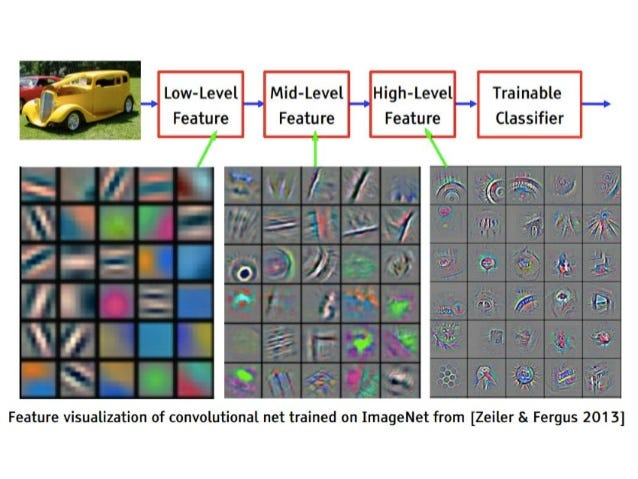Interesting Deep Learning Techniques — Transfer Learning with Resnet and Differential Learning…

This article is in continuation of my first article on interesting deep learning techniques available here. At the end of series, we will apply these techniques to planet-understanding-the-amazon-from-space kaggle competition using the Fast.ai library

Transfer Learning

Transfer learning is a research problem in machine learning that focuses on storing knowledge gained while solving one problem and applying it to a different but related problem. For example, knowledge gained while learning to recognize Imagenet images could apply when trying to recognize planet-understanding-the-amazon-from-space Kaggle competition though they are quite different.

knowledge gained on Imagenet trained model can be seen as below.

Resnet

A deep residual network (deep ResNet) is a type of specialized neural network trained on Imagenet dataset that helps to handle more sophisticated deep learning tasks and models. Resnet bagged all Imagenet competitions like classification, multilabel classification and object localization on kaggle, as such the strength of Resent we gonna make use of this architecture to achieve state of art results in Planet competition.

Differential Learning Rates

The differential Learning rate is an idea of applying different learning rates to different layers by freezing and unfreezing layers, instead of one common learning rate across the network, which makes sense, particularly in transfer learning as the pre-trained model would have learned to identify the initial lower level features which can be reused while applying to the different set of images.

Resnet Convolutional layers all contain pre-trained weights, for things that are close to ImageNet, they are really good; for things that are not close to ImageNet, they are better than nothing. All weights of our fully connected layers which are at the end of Network are totally random. Therefore, you would always want to freeze initial layers and make the fully connected weights better than random by training them a bit first. Otherwise, if you go straight to unfreeze, then you are actually going to be fiddling around with those early layer weights when the later ones are still random — which is probably not what we want.

`#inital train fully connected layers using single learning ratelr = 0.2learn.fit(lr, 3, cycle_len=1, cycle_mult=2)`
`#use differential learning rate, across layerslrs = np.array([lr/9,lr/3,lr])learn.unfreeze()learn.fit(lrs, 3, cycle_len=1, cycle_mult=2)`

Planet Competition

We will make use of the Fast.ai library to solve this problem. The fast.ai library is not only a toolkit to get newbies quickly implementing deep learning, but a powerful and convenient source of current best practices. Each time the fast.ai team (and their network of AI researchers & collaborators) finds a particularly interesting paper, they test it out on a variety of datasets and work out how to tune it. If they are successful, it gets implemented in the library.

`sz=64`
`learn.set_data(get_data(sz))learn.freeze()learn.fit(lr, 3, cycle_len=1, cycle_mult=2)`
`learn.unfreeze()learn.fit(lrs, 3, cycle_len=1, cycle_mult=2)learn.save(f'{sz}')`